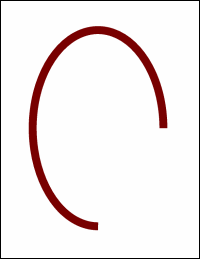AddArc MethodAdds an arc to the current page.Syntax

 ```GraphicID = Doc.AddArc(AngleStart, AngleEnd, CenterX, CenterY, RadiusX, RadiusY [, Filled])```Params

 Name Type Description AngleStart Double The start angle of the arc in degrees. AngleEnd Double The end angle of the arc in degrees. CenterX Double The horizontal coordinate of the center of the arc. CenterY Double The vertical coordinate of the center of the arc. RadiusX Double The horizontal radius of the arc. RadiusY Double The vertical radius of the arc. Filled Boolean Whether to fill the arc rather than simply drawing it (optional). GraphicID Long The Object ID of the newly added Graphic object.Notes

 Adds an arc to the current page. The arc is drawn in the current color at the current width and with the current options. The arc is positioned at the center coordinates and can have distinct horizontal and vertical radii. Drawing starts at the start angle, and the arc is swept out until the end angle is reached. Angles are measured anti-clockwise with zero at three o'clock. The AddArc method returns the Object ID of the newly added Graphic object.Example

 The following code adds an arc to a document. ```Set theDoc = Server.CreateObject("ABCpdf12.Doc") theDoc.Width = 24 theDoc.Color = "120 0 0" theDoc.AddArc 0, 270, 300, 400, 200, 300 theDoc.Save "c:\mypdfs\docaddarc.pdf"```docaddarc.pdf